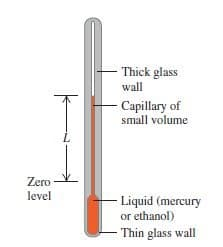Physic Tutorials

# What is temperature and temperature measurement in Physics

The idea of temperature is rooted in qualitative ideas of “hot” and “cold” based on the sense of touch. As we know that an object that feels hot usually has a higher temperature than when it feels cold.We are used to the idea that a thermometer shows the temperature of an object with which is in contact with.

What I will be discussing in this tutorial is listed below

1. What is temperature or how temperature is defined in physics?
2. What are the different units of temperature?
3. Conversion of different temperature scales
4. Temperature measurement
5. Temperature examples
6. Types of thermometer

## What is temperature or how temperature is defined in physics?

Temperature is a measure of degree of hotness or coldness of an object on some scale. You can also say it tells us the direction in which energy flows (energy flow from a region of higher temperature to the region of lower temperature).

When two objects in contact have the same temperature, there will be no transfer of thermal energy between them. This is referred to as thermal equilibrium.

## What are the different units of temperature?

The unit of temperature includes Celsius, Kelvin, and Fahrenheit. However, the S.I base unit of temperature is Kelvin. Measuring temperature in Kelvin scale is better than that of Celsius scale in that one of its fixed points, absolute zero has much significance than the higher or lower fixed points of Celsius scale.

Note: it is impossible to have a temperature lower than zero Kelvin (0 K is absolute zero).

## Conversion of different temperature scales

To convert from Celsius to Kelvin = temperature in Celsius + 273.15

To convert from Kelvin to Celsius = temperature in Kelvin – 273.15

Example

Convert -23 degree Celsius to Kelvin

Solution

-23 + 273.15 = 250.15 K

Note: the triple point of water is the temperature at which ice, water, and water vapour can co-exist. And it is defined as 273.16 K (273.16 – 273.15 = 0.01 C)

To convert from Celsius to Fahrenheit or vice-versa

C/5 = (F-32)/9

C is Celsius scale while F is Fahrenheit scale

Example

Convert 50 degree Celsius to Fahrenheit

50/5 = (F-32)/9

10 = (F-32)/9

10 X 9 = F-32

90 = F -32

F = 90 + 32

F = 122

## Temperature measurement

Temperature of an object is measured with a thermometer.

Types of thermometer

• Resistance thermometer
• Thermocouples
• Mercury-in-glass thermometer
• Clinical thermometer

Differences between Resistance thermometer and Thermocouple

Resistance thermometer is larger than thermocouple, has greater thermal capacity therefore slower acting while thermocouple is smaller, has smaller capacity, therefore quicker acting and can measure temperature at a point.

Recommended

Heat Capacity

Linear Expansivity

### Bolarinwa Olajire

A lecturer, Educationist, PhD student at FUNAAB, and a Blogger.